# EU Long-Term Interest Rates, April 2021

Jun 8, 2021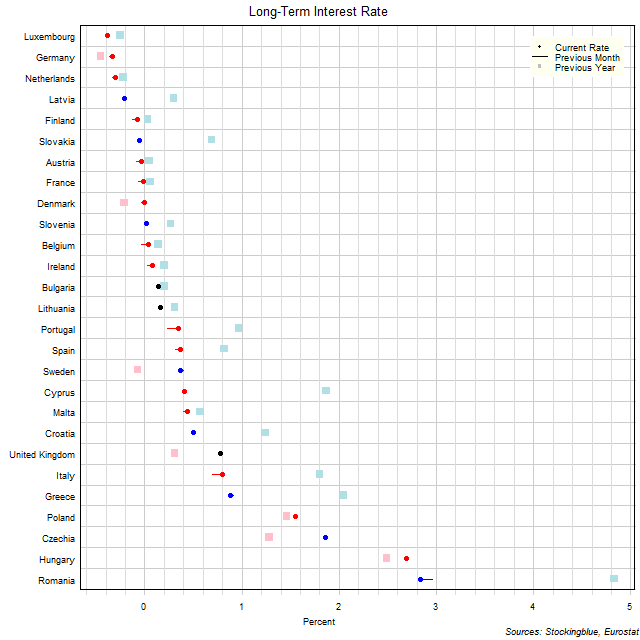The chart above shows the ten-year interest rate in each EU state as of April 2021, the change from the previous month, and the rate one year prior.  Eight states have a negative interest rate (down from 10 last month and up from 5 last year).

# EU Long-Term Interest Rates, March 2021

May 6, 2021The chart above shows the ten-year interest rate in each EU state as of March 2021, the change from the previous month, and the rate one year prior.  Ten states have a negative interest rate (down from 11 last month and same as 10 last year).

# EU Long-Term Interest Rates, February 2021

Apr 8, 2021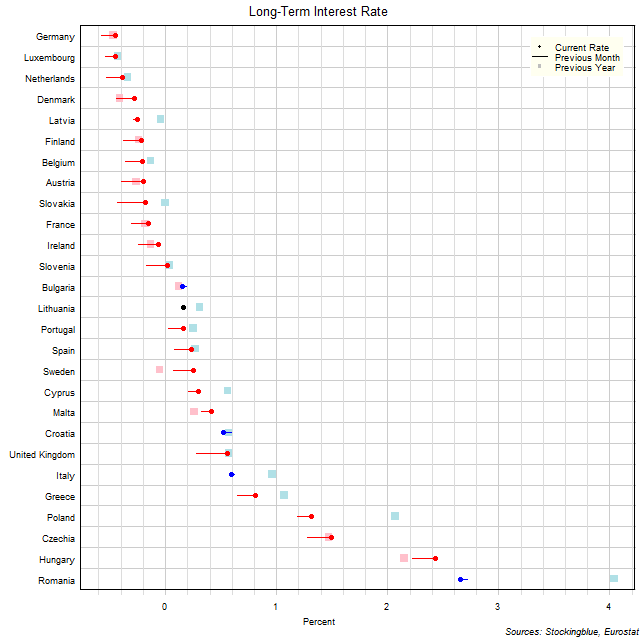The chart above shows the ten-year interest rate in each EU state as of February 2021, the change from the previous month, and the rate one year prior.  Eleven states have a negative interest rate (down from 12 last month and same as 11 last year).

# EU Long-Term Interest Rates, January 2021

Mar 8, 2021The chart above shows the ten-year interest rate in each EU state as of January 2021, the change from the previous month, and the rate one year prior.  Twelve states have a negative interest rate (same as 12 last month and up from eight last year).

# EU Long-Term Interest Rates, December 2020

Feb 3, 2021The chart above shows the ten-year interest rate in each EU state as of December 2020, the change from the previous month, and the rate one year prior.  Twelve states have a negative interest rate (same as 12 last month and up from six last year).

# EU Long-Term Interest Rates, November 2020

Jan 11, 2021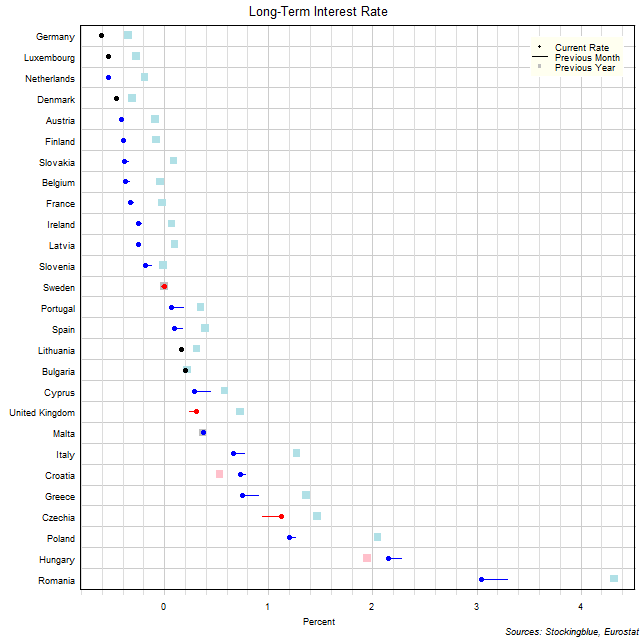The chart above shows the ten-year interest rate in each EU state as of November 2020, the change from the previous month, and the rate one year prior.  Twelve states have a negative interest rate (down from 13 last month and up from nine last year).

# EU Long-Term Interest Rates, October 2020

Dec 8, 2020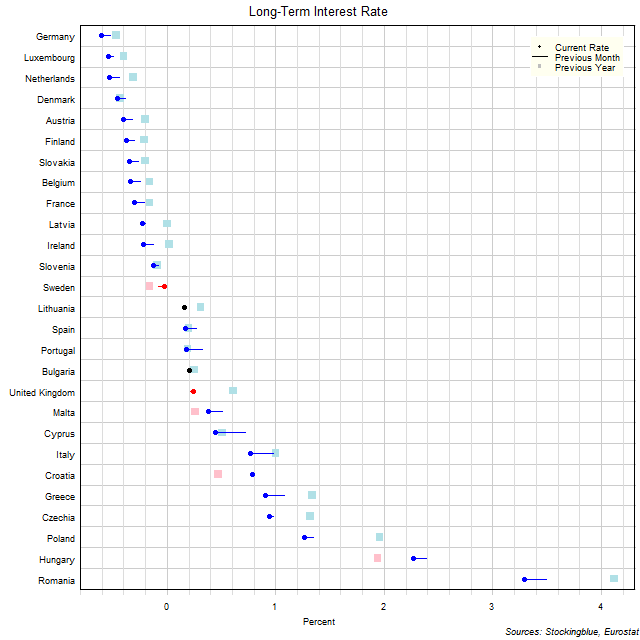The chart above shows the ten-year interest rate in each EU state as of October 2020, the change from the previous month, and the rate one year prior.  Thirteen states have a negative interest rate (same as 13 last month and up from 11 last year).

# EU Long-Term Interest Rates, September 2020

Nov 10, 2020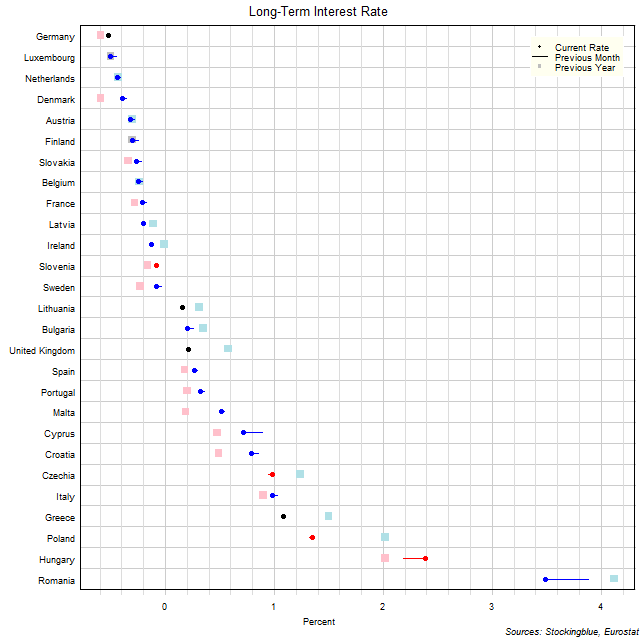The chart above shows the ten-year interest rate in each EU state as of September 2020, the change from the previous month, and the rate one year prior.  Thirteen states have a negative interest rate (same as 13 last month and same as 13 last year).

# EU Long-Term Interest Rates, August 2020

Oct 5, 2020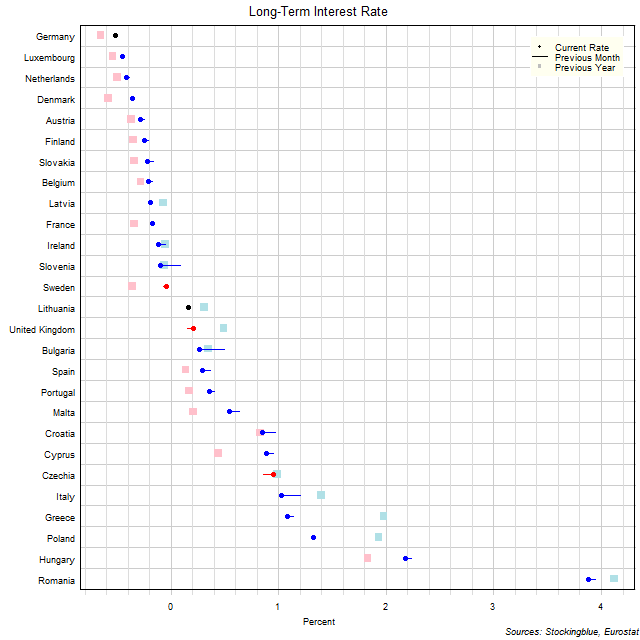The chart above shows the ten-year interest rate in each EU state as of August 2020, the change from the previous month, and the rate one year prior.  Thirteen states have a negative interest rate (up from 12 last month and same as 13 last year).

# EU Long-Term Interest Rates, July 2020

Sep 7, 2020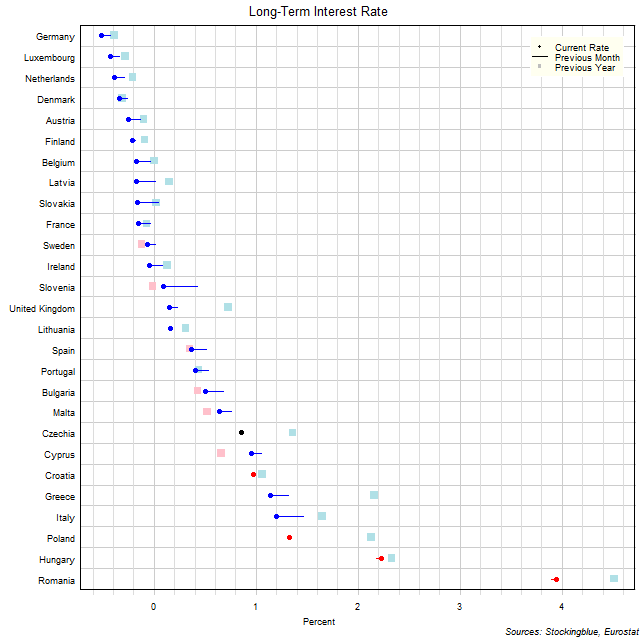The chart above shows the ten-year interest rate in each EU state as of July 2020, the change from the previous month, and the rate one year prior.  Twelve states have a negative interest rate (up from eight last month and up from nine last year).

Older